# Highlight the minimum value in each column In Pandas

In this article, we will discuss how to Highlight the minimum values in Pandas Dataframe. So, Let’s first make a dataframe:

## Python3

 `# Import Required Libraries ` `import` `pandas as pd ` `import` `numpy as np ` ` `  `# Create a dictionary for the dataframe ` `dict` `=` `{ ` `  ``'Name'``: [``'Sumit Tyagi'``, ``'Sukritin'``,  ` `           ``'Akriti Goel'``, ``'Sanskriti'``, ` `           ``'Abhishek Jain'``], ` `  ``'Age'``: [``22``, ``20``, ``45``, ``21``, ``22``], ` `  ``'Marks'``: [``90``, ``84``, ``33``, ``87``, ``82``] ` `} ` ` `  `# Converting Dictionary to  ` `# Pandas Dataframe ` `df ``=` `pd.DataFrame(``dict``) ` ` `  `# Print Dataframe ` `print``(df)`

Output: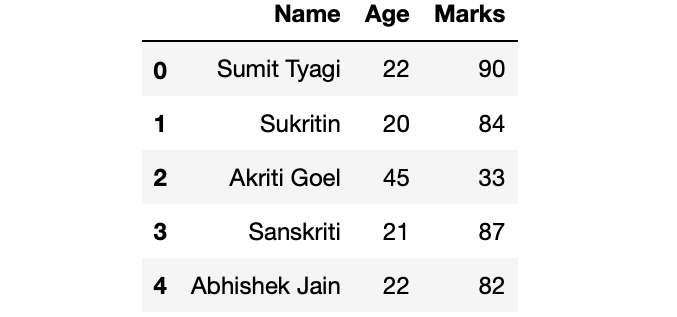Now, come to the highlighting part. Our objective is to highlight cells with minimum values in each column.

Method 1: Using df.style.highlight_min() method.

Syntax: DataFrame.style.highlight_min(subset, color, axis)
Parameters:

• subset: Name of the columns of which you want to find the minimum.
• color: Name of the color with which you want to highlight the cell
• axis: {0 or ‘index’, 1 or ‘columns’} based on which axis you want find the minimum.

Returns: Styler object.

Example: Highlighting Cell with minimum value in each column.

## Python3

 `# Highlighting the minimum values of last 2 columns ` `df.style.highlight_min(color ``=` `'lightgreen'``,  ` `                       ``axis ``=` `0``) `

Output: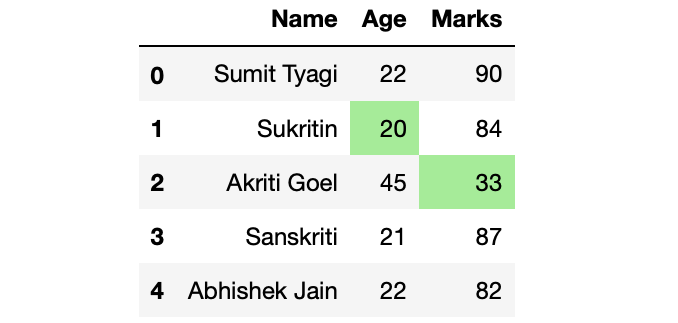Method 2: Using the df.style.apply() method.

Syntax: DataFrame.style.apply(self, func, axis=0, subset=None, **kwargs)
Parameters:

• func: It should take a pandas.Series or pandas.DataFrame based on the axis and should return an object with the same shape.
• axis: {0 or ‘index’, 1 or ‘columns’, None}, default 0. Apply to each column (axis=0 or ‘index’), to each row (axis=1 or ‘columns’), or to the entire Dataframe at once with axis=None.
• subset: Set of columns or rows on which you want to call the func.
• **kwargs: Pass along to func.

Returns: Styler object.

Example 1: Highlighting the text instead of cell.

## Python3

 `# Defining custom function  ` `# which returns the list for ` `# df.style.apply() method ` `def` `highlight_min(s): ` `   `  `    ``is_min ``=` `s ``=``=` `s.``min``() ` `     `  `    ``return` `[``'color: green'` `if` `cell ``else` `''  ` `            ``for` `cell ``in` `is_min] ` ` `  `df.style.``apply``(highlight_min)`

Output: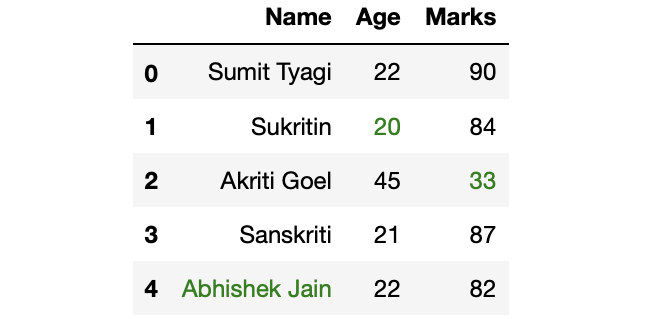Example 2: Highlighting cell with minimum values.

## Python3

 `# Defining custom function ` `# which returns the list for ` `# df.style.apply() method ` `def` `highlight_min(s): ` `    ``is_min ``=` `s ``=``=` `s.``min``() ` `     `  `    ``return` `[``'background: lightgreen'` `if` `cell ``else` `''  ` `            ``for` `cell ``in` `is_min] ` ` `  `df.style.``apply``(highlight_min)`

Output: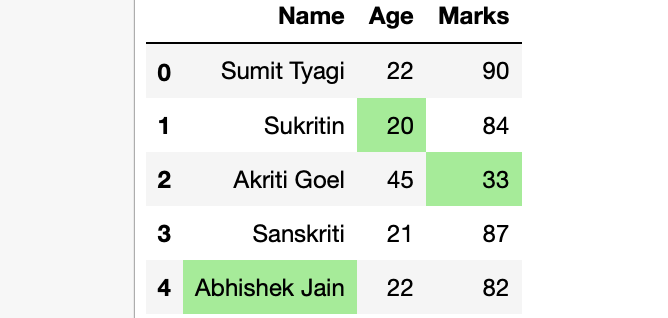Example 3: Highlighting cell with minimum values but not highlighting the string values.

## Python3

 `# Defining custom function  ` `# which returns the list for ` `# df.style.apply() method ` `def` `highlight_min(s): ` `    ``if` `s.dtype ``=``=` `np.``object``: ` `        ``is_min ``=` `[``False` `for` `_ ``in` `range``(s.shape[``0``])] ` `    ``else``: ` `        ``is_min ``=` `s ``=``=` `s.``min``() ` `         `  `    ``return` `[``'background: lightgreen'` `if` `cell ``else` `''  ` `            ``for` `cell ``in` `is_min] ` ` `  `df.style.``apply``(highlight_min)`

Output: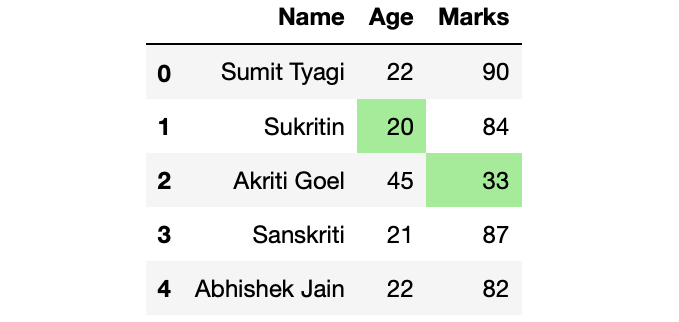My Personal Notes arrow_drop_upCheck out this Author's contributed articles.

If you like GeeksforGeeks and would like to contribute, you can also write an article using contribute.geeksforgeeks.org or mail your article to contribute@geeksforgeeks.org. See your article appearing on the GeeksforGeeks main page and help other Geeks.

Please Improve this article if you find anything incorrect by clicking on the "Improve Article" button below.

Article Tags :

Be the First to upvote.

Please write to us at contribute@geeksforgeeks.org to report any issue with the above content.